Search by Topic

Resources tagged with Working systematically similar to Polynomial Interpolation:

Filter by: Content type:
Age range:
Challenge level:

There are 74 results

Broad Topics > Using, Applying and Reasoning about Mathematics > Working systematicallyInterpolating Polynomials

Age 16 to 18 Challenge Level:

Given a set of points (x,y) with distinct x values, find a polynomial that goes through all of them, then prove some results about the existence and uniqueness of these polynomials.Plum Tree

Age 14 to 18 Challenge Level:

Label this plum tree graph to make it totally magic!W Mates

Age 16 to 18 Challenge Level:

Show there are exactly 12 magic labellings of the Magic W using the numbers 1 to 9. Prove that for every labelling with a magic total T there is a corresponding labelling with a magic total 30-T.All-variables Sudoku

Age 11 to 18 Challenge Level:

The challenge is to find the values of the variables if you are to solve this Sudoku.The Great Weights Puzzle

Age 14 to 16 Challenge Level:

You have twelve weights, one of which is different from the rest. Using just 3 weighings, can you identify which weight is the odd one out, and whether it is heavier or lighter than the rest?Advent Calendar 2011 - Secondary

Age 11 to 18 Challenge Level:

Advent Calendar 2011 - a mathematical activity for each day during the run-up to Christmas.A Long Time at the Till

Age 14 to 18 Challenge Level:

Try to solve this very difficult problem and then study our two suggested solutions. How would you use your knowledge to try to solve variants on the original problem?LCM Sudoku II

Age 11 to 18 Challenge Level:

You are given the Lowest Common Multiples of sets of digits. Find the digits and then solve the Sudoku.Magic W

Age 14 to 16 Challenge Level:

Find all the ways of placing the numbers 1 to 9 on a W shape, with 3 numbers on each leg, so that each set of 3 numbers has the same total.Exhaustion

Age 16 to 18 Challenge Level:

Find the positive integer solutions of the equation (1+1/a)(1+1/b)(1+1/c) = 2Problem Solving, Using and Applying and Functional Mathematics

Age 5 to 18 Challenge Level:

Problem solving is at the heart of the NRICH site. All the problems give learners opportunities to learn, develop or use mathematical concepts and skills. Read here for more information.Games Related to Nim

Age 5 to 16

This article for teachers describes several games, found on the site, all of which have a related structure that can be used to develop the skills of strategic planning.Age 11 to 16 Challenge Level:

Four numbers on an intersection that need to be placed in the surrounding cells. That is all you need to know to solve this sudoku.Star Product Sudoku

Age 11 to 16 Challenge Level:

The puzzle can be solved by finding the values of the unknown digits (all indicated by asterisks) in the squares of the $9\times9$ grid.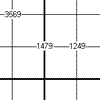Another Quadruple Clue Sudoku

Age 11 to 16 Challenge Level:

This is a variation of sudoku which contains a set of special clue-numbers. Each set of 4 small digits stands for the numbers in the four cells of the grid adjacent to this set.Diagonal Product Sudoku

Age 11 to 16 Challenge Level:

Given the products of diagonally opposite cells - can you complete this Sudoku?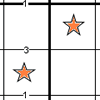Constellation Sudoku

Age 14 to 18 Challenge Level:

Special clue numbers related to the difference between numbers in two adjacent cells and values of the stars in the "constellation" make this a doubly interesting problem.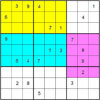Colour Islands Sudoku 2

Age 11 to 18 Challenge Level:

In this Sudoku, there are three coloured "islands" in the 9x9 grid. Within each "island" EVERY group of nine cells that form a 3x3 square must contain the numbers 1 through 9.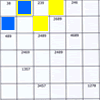Intersection Sudoku 2

Age 11 to 16 Challenge Level:

A Sudoku with a twist.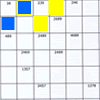Intersection Sudoku 1

Age 11 to 16 Challenge Level:

A Sudoku with a twist.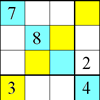Rectangle Outline Sudoku

Age 11 to 16 Challenge Level:

Each of the main diagonals of this sudoku must contain the numbers 1 to 9 and each rectangle width the numbers 1 to 4.Warmsnug Double Glazing

Age 14 to 16 Challenge Level:

How have "Warmsnug" arrived at the prices shown on their windows? Which window has been given an incorrect price?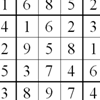Twin Chute-swapping Sudoku

Age 14 to 18 Challenge Level:

A pair of Sudokus with lots in common. In fact they are the same problem but rearranged. Can you find how they relate to solve them both?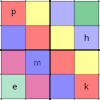Simultaneous Equations Sudoku

Age 11 to 16 Challenge Level:

Solve the equations to identify the clue numbers in this Sudoku problem.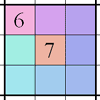Integrated Sums Sudoku

Age 11 to 16 Challenge Level:

The puzzle can be solved with the help of small clue-numbers which are either placed on the border lines between selected pairs of neighbouring squares of the grid or placed after slash marks on. . . .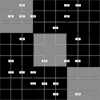Ratio Sudoku 2

Age 11 to 16 Challenge Level:

A Sudoku with clues as ratios.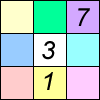Age 11 to 16 Challenge Level:

Four small numbers give the clue to the contents of the four surrounding cells.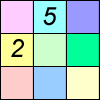Difference Sudoku

Age 14 to 16 Challenge Level:

Use the differences to find the solution to this Sudoku.An Introduction to Magic Squares

Age 7 to 16

Find out about Magic Squares in this article written for students. Why are they magic?!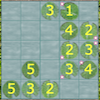Latin Lilies

Age 7 to 16 Challenge Level:

Have a go at this game which has been inspired by the Big Internet Math-Off 2019. Can you gain more columns of lily pads than your opponent?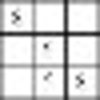Twin Corresponding Sudokus II

Age 11 to 16 Challenge Level:

Two sudokus in one. Challenge yourself to make the necessary connections.Pole Star Sudoku 2

Age 11 to 16 Challenge Level:

This Sudoku, based on differences. Using the one clue number can you find the solution?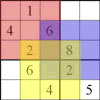Seasonal Twin Sudokus

Age 11 to 16 Challenge Level:

This pair of linked Sudokus matches letters with numbers and hides a seasonal greeting. Can you find it?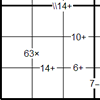Bochap Sudoku

Age 11 to 16 Challenge Level:

This Sudoku combines all four arithmetic operations.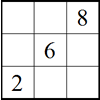Twin Corresponding Sudoku III

Age 11 to 16 Challenge Level:

Two sudokus in one. Challenge yourself to make the necessary connections.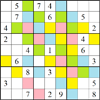Rainstorm Sudoku

Age 14 to 16 Challenge Level:

Use the clues about the shaded areas to help solve this sudokuDifference Dynamics

Age 14 to 18 Challenge Level:

Take three whole numbers. The differences between them give you three new numbers. Find the differences between the new numbers and keep repeating this. What happens?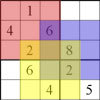Twin Corresponding Sudoku

Age 11 to 18 Challenge Level:

This sudoku requires you to have "double vision" - two Sudoku's for the price of one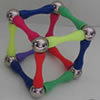Magnetic Personality

Age 7 to 16 Challenge Level:

60 pieces and a challenge. What can you make and how many of the pieces can you use creating skeleton polyhedra?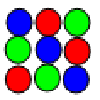Latin Squares

Age 11 to 18

A Latin square of order n is an array of n symbols in which each symbol occurs exactly once in each row and exactly once in each column.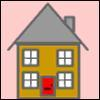Smith and Jones

Age 14 to 16 Challenge Level:

Mr Smith and Mr Jones are two maths teachers. By asking questions, the answers to which may be right or wrong, Mr Jones is able to find the number of the house Mr Smith lives in... Or not!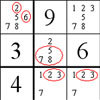The Naked Pair in Sudoku

Age 7 to 16

A particular technique for solving Sudoku puzzles, known as "naked pair", is explained in this easy-to-read article.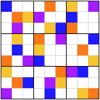Pole Star Sudoku

Age 14 to 18 Challenge Level:

A Sudoku based on clues that give the differences between adjacent cells.Colour in the Square

Age 7 to 16 Challenge Level:

Can you put the 25 coloured tiles into the 5 x 5 square so that no column, no row and no diagonal line have tiles of the same colour in them?Age 11 to 16 Challenge Level:

The items in the shopping basket add and multiply to give the same amount. What could their prices be?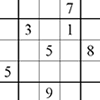Corresponding Sudokus

Age 11 to 18

This second Sudoku article discusses "Corresponding Sudokus" which are pairs of Sudokus with terms that can be matched using a substitution rule.LCM Sudoku

Age 14 to 16 Challenge Level:

Here is a Sudoku with a difference! Use information about lowest common multiples to help you solve it.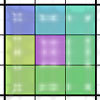Diagonal Sums Sudoku

Age 7 to 16 Challenge Level:

Solve this Sudoku puzzle whose clues are in the form of sums of the numbers which should appear in diagonal opposite cells.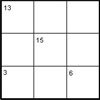Intersection Sums Sudoku

Age 7 to 16 Challenge Level:

A Sudoku with clues given as sums of entries.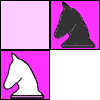Knights Moving

Age 16 to 18 Challenge Level:

Can you swap the black knights with the white knights in the minimum number of moves?